1. Biomedical background and objectives
The research in this proposal has been motivated by a study of blood flow through compliant vessels after endovascular repair. The PI and cardiologist Dr. Krajcer at St. Luke's Episcopal Hospital in Houston have begun a study of blood flow through the abdominal aorta after the insertion of a prosthesis (a spring-like device called stent) used to treat abdominal aneurysm (sac-like protrusion of weakened sections of the aorta). See Figure 1. The procedure entails inserting a stent inside the aneurysm where it serves to hold open the weakened artery and to exclude the aneurysm from circulation. The main goal of this collaborative study is to understand the behavior of the prosthesis and of the walls of the aorta subject to the pressures induced by pulsatile blood flow.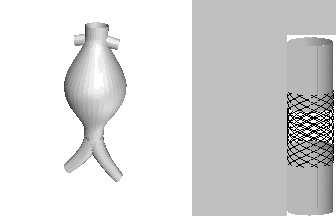A sketch of abdominal aneurysm (left) and a sketch of a stent
inserted in the abdominal aorta (right), not showing the aneurysm sac

The model equations are obtained using asymptotic reduction of the axi-symmetric Navier-Stokes equations in narrow and long channels. The equations have first been derived in , and a mathematically rigorous asymptotic reduction is presented in . The equations are in the form of a quasilinear hyperbolic system of partial differential equations describing conservation of mass and momentum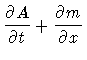= 0, (1)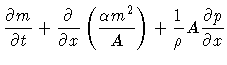= f(A,m). (2)

Here A(x,t) is the cross-sectional area of the vessel, m(x,t) = AV is the momentum, V=V(x,t) is the average axial velocity and p is the transmural pressure. The coefficient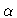accounts for the fact that averaged quantities (momentum) are conserved. The source term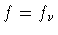includes the effects of viscosity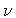via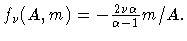To close the system, the pressure term needs to be specified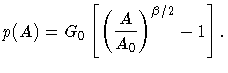(3)

G0 describes tissue stiffness (pressure-strain elastic modulus), anddescribes linear/nonlinear behavior of the channel wall (pressure-strain relationship). Versions of this model have been used by many authors to model fluid flow in compliant tubes [1, 2, 4, 5, 6, 7, 8, 11, 15, 18]. A novel approach in this study is to assume that the coefficient G0 is a piecewise constant function of x to reflect the fact that the stent and the aorta have different elastic properties [20, 14]. As a consequence, stent and the aorta respond differently to the pressure induced by the pulsatile blood flow (see movies). Various complications associated with this phenomenon have been reported in patient studies . The main objective of the present study is to analyze the abrupt changes in the cross-sectional area due to the abrupt changes in the elasticity coefficient G0, understand their consequences on the blood flow, and study optimal design of multiple overlapping stents in order to minimize harmful effects caused by the perturbed channel wall dynamics.

2. Mathematical background and objectives
In order to study discontinuities in the cross-sectional area we need to be able to define a weak solution for a quasilinear system of hyperbolic equations with discontinuous coefficients. There is no general theory that deals with systems of hyperbolic partial differential equations with discontinuous coefficients. The literature is sparse even for hyperbolic equations in conservation form allowing discontinuous coefficients. There are various difficulties associated with the solutions of such systems. For example, physically reasonable solutions exhibit jump discontinuities that are not compressive because the jump discontinuity in the solution is induced by the discontinuity in the coefficients, and not by the compressive nature of the characteristics. Deriving the correct'' entropy criterion for such jump discontinuities, and capturing such solutions numerically, is a problem. In the case when the equations are not in conservation form, as in the case of our model, even the basic issue such as the meaning of the weak form of the equations in unclear. The main difficulty stems from the fact that the weak form of the equations contains the products of the Dirac measure by the Heaviside function which cannot be defined in the sense of distributions.

In the case when the equations are linear and in conservation form there is a theory developed in  that deals with numerical approximation of one-dimensional linear conservation equations with discontinuous coefficients. Unfortunately, the techniques used in  do not generalize to nonlinear problems. Quasilinear scalar hyperbolic problems have been studied in [16, 17] where variational methods were used to study existence and uniqueness of solutions. The methods heavily rely on the fact that there is only one hyperbolic equation under consideration and it is unlikely that the approach can be generalized to systems. Finally, the most relevant to this proposal are the work of A.I Volpert  and a work by Dal Maso, P.G. LeFloch and F. Murat  where issues related to the product of the Dirac measure by the Heaviside function are discussed. Although these works do not deal with hyperbolic problems with discontinuous coefficients, the ideas about the meaning of the ambiguous products are very much related to the problems that arise in hyperbolic equations with discontinuous coefficients. In the work proposed here one of the objectives is to generalize those ideas to the systems with discontinuous coefficients.

For the past ten years the PI has had continuous research support by the National Science Foundation to study properties of solutions to multi-dimensional systems of conservation laws and to study uniqueness and properties of viscosity solutions to one-dimensional conservation laws with smooth coefficients. The project described in this proposal opens a new direction in the PI's research program. Various aspects of this project, however, rely heavily on the knowledge and the experience gained from the work supported by the NSF.

B. Methodology

1. Theoretical approach
To study weak solutions of equations (1) and (2) we begin with the standard approach: multiply the equations by a test function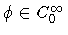and integrate by parts to obtain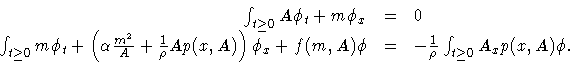(4)

It was shown in  that at the points where the coefficient G0 of p(x,A) is discontinuous, the cross-sectional area Acannot be continuous. This implies that the right hand-side contains the product of the Dirac measure Ax by the Heaviside function p(x,A). This difficulty cannot be avoided if system (1), (2) is written in conservation form. Converting the system into a conservation law gives rise to a source term which is singular; it is the product of a function of A, call it q(A), and the derivative of the discontinuous coefficient, G0'(x), . The weak form of the equations then contains the term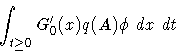(5)

which is not defined in the sense of distributions.

To get around this difficulty we take the following approach. Motivated by the underlying application in which the Young's modulus coefficient G0 is actually continuous, with high gradients at the anchoring cites of the stent, we regularize the problem by smoothing out the Dirac measure term G0'. We regularize Dirac measure by using, the so called, admissible averaging kernels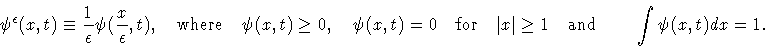Consider the weak form that corresponds to the regularized coefficient G0', call it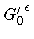, and define the weak form of the original, singular problem to be the one obtained in the limit, as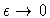, of the regularized forms. In order for the limit to be defined, we need to specify the term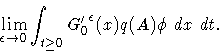(6)

Following the ideas presented in [12, 21], we define (6) to be the mean value of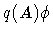with respect to the measure G0' approximated by the admissible averaging kernels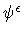, [4, 21]. It can be shown that if the kernel is symmetric, i.e., if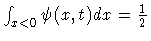, then the resulting symmetric mean does not depend on the choice of the symmetric kernel [4, 21]. The choice of the kernel will depend on the particular problem at hand, and here is where the physics'' of the problem needs to be taken into account in order to recover the physically'' or physiologically reasonable solution''. Although this is similar to the viscosity solutions approach in the theory of conservation laws, the underlying issues of convergence and stability require different techniques. One of the goals of this project is to generalize the techniques presented in  to study convergence and stability of the products like (6) arising in systems with discontinuous coefficients.

2. Numerical simulations
The PI has studied the performance of two numerical methods on the calculation of the solutions of equations (1) and (2) with discontinuous G0: the Lax-Friedrichs method (LF) and the Richmyer two-step Lax-Wendroff method (LW), . Unusual observations have been obtained. The LW-method produces the solution which would have been recovered if symmetric kernels were used to approximate the Dirac measure G0' in the quasilinear form of the problem. The LF-method, on the other hand, produces a different solution from the LW-method, regardless whether one solves the conserved or the nonconserved form of the equations. A group of undergraduate students who participated in the NSF-sponsored REU program this summer, studied the behavior of the LF method. They have shown that the modified equation contains large (artificial) source terms due to the large gradients of G0 and the error contaminates the solution everywhere downstream from the locus of discontinuity. Even when G0 is smoothed out, careful analysis is needed prior to the numerical simulation in order to keep the effects of numerical diffusion below the effects of blood viscosity, described by the source term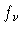, and below the smoothing effects of the discontinuous coefficient G0. Similar analysis needs to be performed before using the second-order LW-method. The issues here are related to balancing the effects of numerical dispersion, numerical diffusion and large artificial source terms, with the regularization of G0 and with the source term.

In this project the PI plans to investigate the effects that need to be taken into account when hyperbolic equations with discontinuous coefficients and singular source terms are simulated in order to obtain the physically reasonable solutions. Error analysis will be performed by generalizing the ideas presented in  to the systems with discontinuous coefficients.

3. Schedule
Although the development of a general existence and uniqueness theory for quasilinear hyperbolic problems with discontinuous coefficients is one of the goals of the PI's research, it is unrealistic to expect that within the two years of the duration of this grant the PI and the students will be able to complete the theory. A realistic goal is to study equations (1) and (2) and gain an insight into the properties of the solutions that can be generalized to a larger class of equations.

C. Research personnel

The PI on the project is Canic. She has extensive research experience in hyperbolic conservation laws, in conservation laws that change type, and in modeling of physical processes by conservation laws. Her accomplishments have been recently recognized by the UH 2001 Associate Professor Research and Scholarship Award. The PI has experience in mentoring undergraduate students (3), graduate students (2) and post-doctoral visitors (1). In addition, this summer she ran a Research for Undergraduates program at UH. One of the students (Burns) who participated in the program is expected to graduate in the Fall and continue working on a problem related to this project as graduate student in Mathematics. Another student (Sharma), currently a graduate student in Mechanical Engineering, is expected to change majors and begin working with the PI on the numerical simulation and error analysis of the model equations with discontinuous G0.

D. Institutional commitment and sources of additional support

The Mathematics Department employs graduate students as Teaching Fellows, and this would be the normal source of support for students engaged in the project. The requested budget will partially support two graduate students providing reduced teaching load to expedite the time to degree. The students have already been recruited. The PI has directed students who are now employed in academia and in industry. In addition, the Mathematics Department is planning to submit a VIGRE (Vertical Integration of Research and Education) proposal in Fall 2001. If awarded, the VIGRE support would provide supplemental funds for two graduate students and one postdoctoral visitor.

The PI has external support which expires this summer. A renewal for the grant will be submitted in the Fall. If awarded, the grant will provide partial travel support and partial summer salary.

E. Student involvement and training opportunities in science and engineering

From the very beginning of this project students and postdoctoral visitors have been involved in numerical simulation, visualization, and the development of a new theory to study the underlying model equations. Two undergraduate students graduated (Pritts, Roy) and continued their studies at the first rate universities. Post-doctoral visitor Kim is moving to a tenure-track position to continue her career in teaching and research. In addition, a group of the most talented undergraduate students from all over the US has been involved this summer in the NSF-sponsored REU program. The topics of their projects originated from the blood-flow problem described here. All of them will continue their studies as graduate students. Student problems that cover a broad range of interests have been formulated and offered by the PI to the graduate student community at UH (two students have already been recruited).

Student project 1. Analysis of weak solutions to conservation laws with discontinuous coefficients and a study of stability of the related singular products with the focus on the blood flow problem.

Student project 2. Numerical simulation of equations (1) and (2) with discontinuous coefficient G0, using different numerical methods. Analysis of the influence of the artifical numerical effects on the calculation of the solution. Error analysis.

Student project 3.
Modeling on the behavior of the prostheses. Development of a more sophisticated model and comparison with the measurements obtained by Dr. Chandar at UT Austin. Inclusion of the porosity of the stent in the model equations.

Student project 4.
Development of a simplified two-dimensional model through curved vessels. This entails studying fluid-structure interactions between the stent/aorta and the blood flow.